# Family

Martin has just as brothers as sisters. His sister Jana but has 2 times more brothers than sisters.

a) How many children are in this family?
b) How many boys and how many girls are in the family?

Correct result:

a =  7
b =  4
c =  3

#### Solution:

a = b+c
b -1 = c
2(c - 1) = b

a = b+c
b -1 = c
2•(c - 1) = b

a-b-c = 0
b-c = 1
b-2c = -2

a = 7
b = 4
c = 3

Our linear equations calculator calculates it.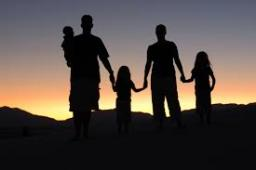We would be pleased if you find an error in the word problem, spelling mistakes, or inaccuracies and send it to us. Thank you!Tips to related online calculators
Do you have a linear equation or system of equations and looking for its solution? Or do you have quadratic equation?

## Next similar math problems:

• Brothers and sistersLenka has as many brothers as sisters. Her brothers have twice as many sisters as brothers. How many brothers and sisters are in the family?
• Large familyI have as many brothers as sisters and each my brother has twice as many sisters as brothers. How many children do parents have?
• Brothers and sisters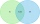There are 35 children in the class, 23 of them have a brother, and 27 of them have a sister. How many children have both a brother and a sister when there are 5 children in the class who have no brother or sister?
• ChildrenThe group has 42 children. There are 4 more boys than girls. How many boys and girls are in the group?
• School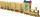School attend 792 children, boys and girls ratio is 4:5. How many more girls go to school (than boys)?
• Boys and girlsThere are 48 children in the sports club, boys are 10 more than girls. How many girls go to the club?
• Family and age ratiosAge of father and son are in the ratio 10:3; age of father and daughter are in the ratio 5:2. How old is father and son, if daughter is 20 years old? In what ratio is age sister and brother?
• Classroom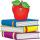In a classroom are 30 students, boys are four times more than girls. How many boys are in the class?
• Glasses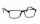There are 36 pupils in the class. Nine girls wear glasses. Boys with glasses are five less than girls without glasses. Boys without glasses are two times more than girls without glasses. How many boys and how many girls?
• Classroom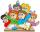In a class are 32 pupils. Boys are 8 less than girls. How many boys and girls are in the classroom?
• The store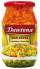The store received the same number of cans of peas and corn. The first day sold 10 cans of peas and 166 cans ofcorn so that left 5 times more peas than corn cans. How many cans of each kind were in the store?
• Cents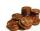Julka has 3 cents more than Hugo. Together they have 27 cents. How many cents has Julka and how many Hugo?
• Students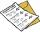The class has 22 students. 2 of which has assesement of 2. Count of assesement of 1 is three times more than count of students with assesement of 3. How many students has assesement of 1 and how many students has assesement of 1.
• Two sisters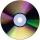Two sisters together have 54 CDs. 6/8 CDs has younger sister and is equal to 3/5 of CDs older sisters. How many CDs has each of the sisters?
• Boys and girls 2The ratio of boys to girls in math club is 4:3 . After 8 more girls joined the Club, the ratio become 1:1. How many members are there in the club now?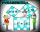Two fifth-graders teams competing in math competitions - in Mathematical Olympiad and Pytagoriade. Of the 33 students competed in at least one of the contest 22 students. Students who competed only in Pytagoriade was twice more than those who just compete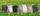In the hutch are 48 mottled rabbits. Brown are 23 less than mottled and white are 8-times less than mottled. How many rabbits are in the hutch?